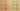EqualityOperatorComparesObjectsValues

Misconception:

The equality operator == compares two objects’ values, for example producing true if they contain the same values for every field.Incorrect

o==p compares the objects referred to by variables o and p

Correct

o==p compares the references stored in the variables o and p

CorrectionHere is what's right.

The Java operator == checks for reference equality, that is it checks whether its left and right operands refer to the same object.

Integer a = new Integer(1);
Integer b = new Integer(1);
assert !(a == b); // a and b are NOT the same

If the equality operator == compared objects looking at their values, it would conclude that a is equal to b (the two objets both contain an instance variable storing the value 1). But that is not what happens: the comparison is made between the two references contained in the two variables. Because the two references refer to two distinct objects in memory, the comparison returns false.

OriginWhere could this misconception come from?

When they start to learn how to program, students usually only deal with primitive values, that are indeed directly comparable using the == operator.

That knowledge might be improperly transferred to complex data structures and/or objects.

SymptomsHow do you know your students might have this misconception?

Students use the == operator to compare objects’ state.

They don’t know the existence of an equals method in the Object class, they have no clue about when it should be used, and they never implement an equals method for the classes they write.

ValueHow can you build on this misconception?

This misconception is a good opportunity to discuss the difference between the general concepts of structural equality (equal objects, i.e., two objects with equivalent state) and referential equality (same object).

Some languages, e.g., Kotlin, provide separate operators for the two kinds of equality. Java only provides an operator for reference equality (==) and expects developers to call the equals(Object) method to test for structural equality.

Java